吴恩达《神经网络和深度学习》课程笔记（3）-- 神经网络基础之Python与向量化1. Vectorization

import numpy as np
import time

a = np.random.rand(1000000)
b = np.random.rand(1000000)

tic = time.time()
c = np.dot(a,b)
toc = time.time()

print(c)
print("Vectorized version:" + str(1000*(toc-tic)) + "ms")

c = 0
tic = time.time()
for i in range(1000000):
c += a[i]*b[i]
toc = time.time()

print(c)
print("for loop:" + str(1000*(toc-tic)) + "ms")


250031.204952
Vectorized version:1.09100341796875ms
250031.204952
for loop:482.65600204467773ms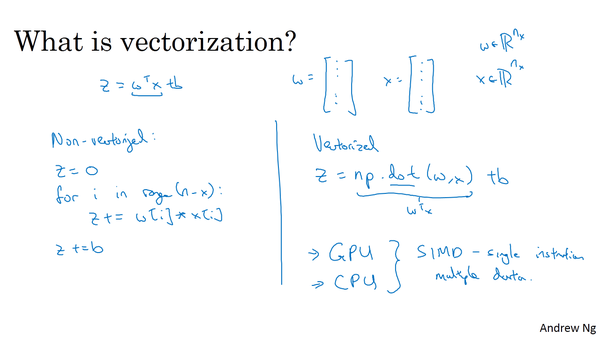2. More Vectorization Examples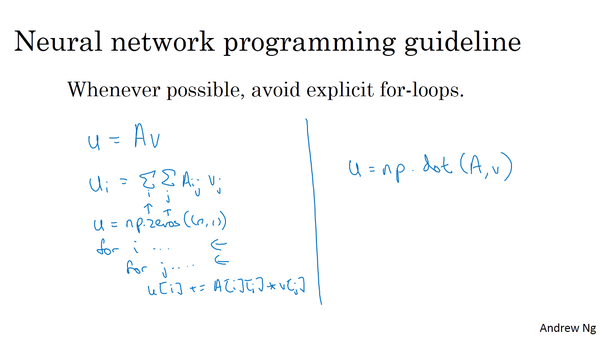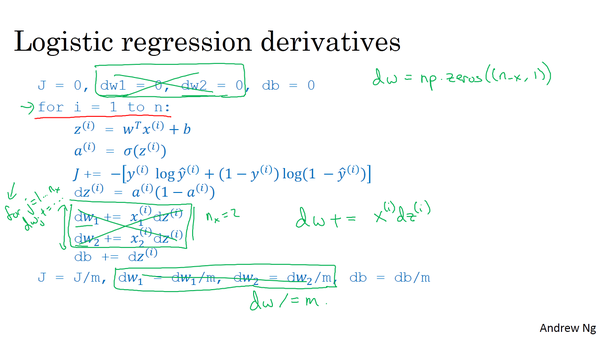3. Vectorizing Logistic RegressionZ = np.dot(w.T,X) + b
A = sigmoid(Z)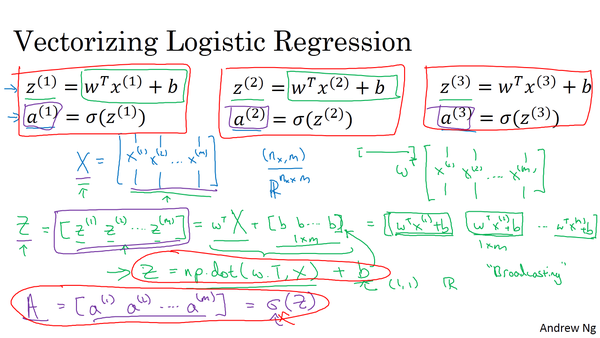4. Vectorizing Logistic Regression’s Gradient Outputdb可表示为：db = 1/m*np.sum(dZ)


dw可表示为：dw = 1/m*np.dot(X,dZ.T)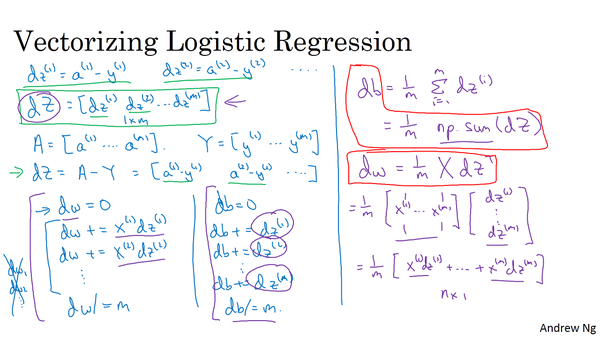Z = np.dot(w.T,X) + b
A = sigmoid(Z)
dZ = A-Y
dw = 1/m*np.dot(X,dZ.T)
db = 1/m*np.sum(dZ)

w = w - alpha*dw
b = b - alpha*db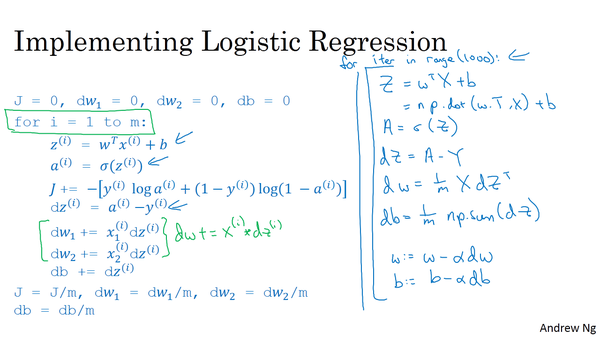• 让所有输入数组都向其中shape最长的数组看齐，shape中不足的部分都通过在前面加1补齐
• 输出数组的shape是输入数组shape的各个轴上的最大值
• 如果输入数组的某个轴和输出数组的对应轴的长度相同或者其长度为1时，这个数组能够用来计算，否则出错
• 当输入数组的某个轴的长度为1时，沿着此轴运算时都用此轴上的第一组值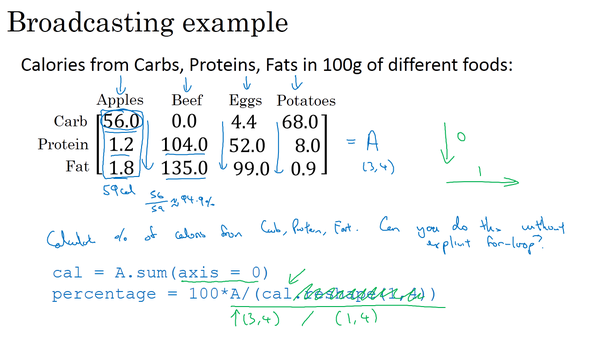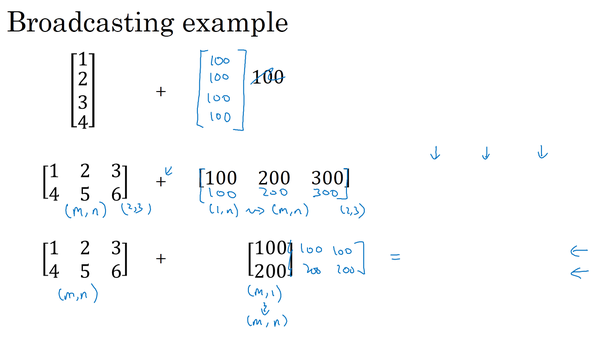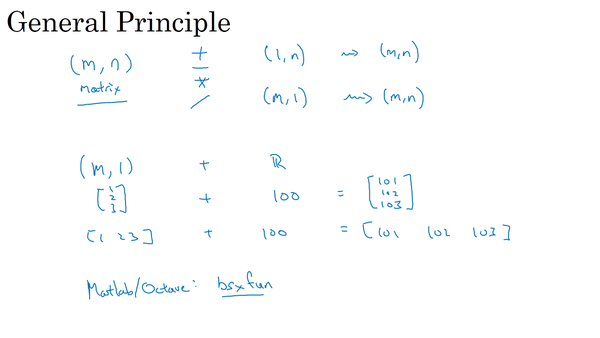6. A note on python/numpy vectors

python中，如果我们用下列语句来定义一个向量：

a = np.random.randn(5)


a = np.random.randn(5,1)
b = np.random.randn(1,5)


assert(a.shape == (5,1))


assert会对内嵌语句进行判断，即判断a的维度是不是（5，1）的。如果不是，则程序在此处停止。使用assert语句也是一种很好的习惯，能够帮助我们及时检查、发现语句是否正确。

a.reshape((5,1))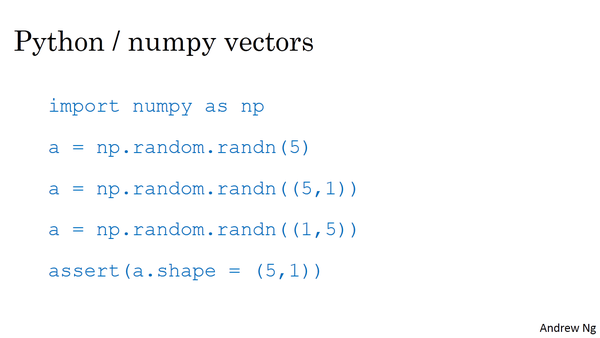7. Quick tour of Jupyter/iPython Notebooks

Jupyter notebook（又称IPython notebook）是一个交互式的笔记本，支持运行超过40种编程语言。本课程所有的编程练习题都将在Jupyter notebook上进行，使用的语言是python。

8. Explanation of logistic regression cost function(optional)由于log函数的单调性，可以对上式P(y x)进行log处理：我们希望上述概率P(y x)越大越好，对上式加上负号，则转化成了单个样本的Loss function，越小越好，也就得到了我们之前介绍的逻辑回归的Loss function形式。Changelog

• 2017-09-24 创建